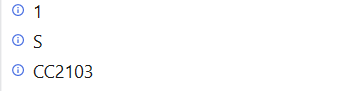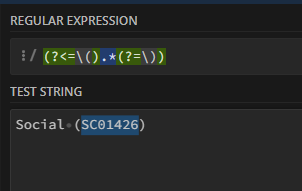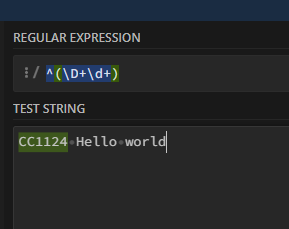# Filter and Extract values from excel rows

FCR.xlsx (12.8 KB)
In attached excel for a Supplier name “RGD” I want to extract the “Spend Category(SC)” and “Cost Center(CC)” value as SC01438 and CC2103 from the rows and there is another default value of “Region” as “USA”

Basically spend category must be the value inside the brackets “()” and cost center ‘the first word of cost center category’ i.e CC2103.

Incase supplier name is not found in the list the values of SC and CC must be as below :
SC - Multi Region
CC - Supplies
Region - USA

Var1(Int32)=DT.Asenumerable.Count(Function(r) r(“Supplier Name”).Tostring.equals(“RGD”))

If
Var1>0
Then

DT1=DT.Asenumerable.Where(Function(r) r(“Supplier Name”).Tostring.equals(“RGD”)).Copytodatatable

Str1= Regex.Match(DT1(0)(“Spend Category”).Tostring,"((.*?))).Group(1).Value

Str2= Regex.Match(DT1(0)(“Cost Centre”).Tostring,“+”).Value

Str3=“USA”

1. 0-9A-Z ↩︎

Hey ,

Thank you for the response.
Str1 is not giving the proper output . It is giving only “S”.Regards,
Gokul

Updated the Query Now Try it Once …And let me Know if you face any challenges

I am getting the below error -

Regards,
GokulTo get the Spend Category

``````System.Text.RegularExpressions.Regex.Match(YourString,"(?<=\().*(?=\))").Tostring
``````To get the Cost Center

``````System.Text.RegularExpressions.Regex.Match(YourString,"^(\D+\d+)").Tostring
``````Regards
Gokul

1 Like

This topic was automatically closed 3 days after the last reply. New replies are no longer allowed.